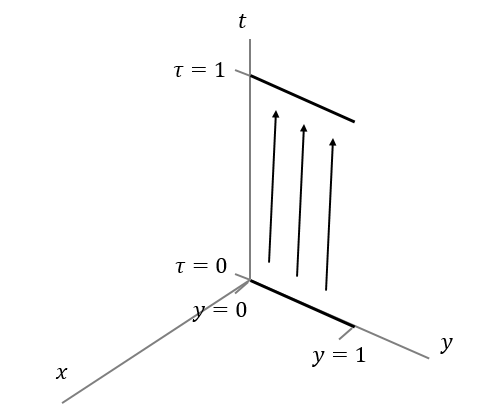# String Worldsheet Area

Consider a relativistic string lying on the y-axis at time $\tau = 0$, stretching from $y=0$ to $y=1$. The string is parameterized so that one endpoint is at $\sigma=0$ and the other is at $\sigma=1$, and so that $\tau = t$. Now allow the string to propagate from time $\tau = 0$ to time $\tau = 1$, leaving the string fixed on the y-axis. Find the area of the resulting string worldsheet.×